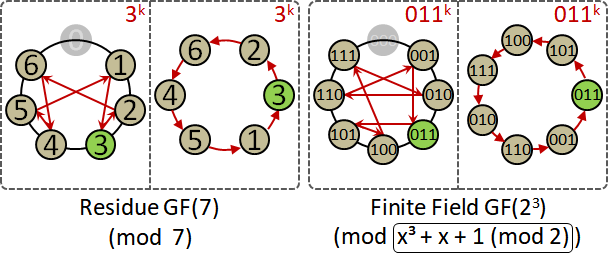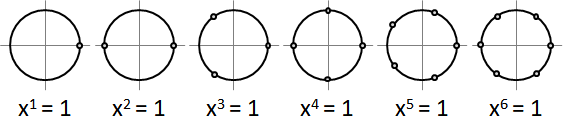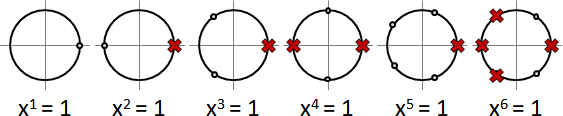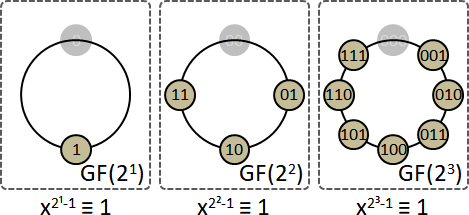Finite Field

Residue Tuple

```(a₀ (mod m₀), a₁ (mod m₁), a₂ (mod m₂))
```

```(a₀, a₁, a₂) (mod m)
```

```(a₀ (mod m₀), a₁ (mod m₁), a₂ (mod m₂))  ←—→  a (mod m₀m₁m₂)
when mᵢ⊥mⱼ
```

```(a₀, a₁, a₂) (mod p)  ←—→  a (mod p³)
```

```(a₀, a₁, a₂) (mod p)  ←—→  a₀x⁰ + a₁x¹ + a₂x² (mod p) (mod p³)
```

Finite Field（Galois Field）

```5 ≡ 2 (mod 3)
4 + 5 ≡ 0 (mod 3)
4 × 5 ≡ 2 (mod 3)
```

```x³ ≡ -x - 1 (mod x³ + x + 1)
x² + (x² + 1) ≡ 2x² + 1 (mod x³ + x + 1)
x² × (x² + 1) ≡ -x (mod x³ + x + 1)
```

```x³ (mod 2) ≡ x + 1 (mod 2) (mod x³ + x + 1 (mod 2))
x² (mod 2) + x² + 1 (mod 2) ≡ 1 (mod 2) (mod x³ + x + 1 (mod 2))
x² (mod 2) × x² + 1 (mod 2) ≡ x (mod 2) (mod x³ + x + 1 (mod 2))
```

```GF(2³), irreducible polynomial is x³ + x + 1 (mod 2)
x³ ≡ x + 1
x² + (x² + 1) ≡ 1
x² × (x² + 1) ≡ x
```

「模數是質數暨質式」暨加減乘除法，數學家稱作「有限體」。

```x (mod 2)          --->  10₍₂₎ = 2₍₁₀₎
x² + 1 (mod 2)     ---> 101₍₂₎ = 5₍₁₀₎
x² + x + 1 (mod 2) ---> 111₍₂₎ = 7₍₁₀₎
```

```GF(2³), irreducible polynomial is x³ + x + 1 (mod 2)
x³ ≡ x + 1         ---> 8 ≡ 3
x² + (x² + 1) ≡ 1  ---> 4 + 5 ≡ 1
x² × (x² + 1) ≡ x  ---> 4 × 5 ≡ 2

+ | 0  1  2  3　4  5  6  7     × | 0  1  2  3　4  5  6  7
--|-----------------------     --|-----------------------
0 | 0  1  2  3  4  5  6  7     0 | 0  0  0  0  0  0  0  0
1 | 1  0  3  2  5  4  7  6     1 | 0  1  2  3  4  5  6  7
2 | 2  3  0  1  6  7  4  5     2 | 0  2  4  6  3  1  7  5
3 | 3  2  1  0  7  6  5  4     3 | 0  3  6  5  7  4  1  2
4 | 4  5  6  7  0  1  2  3     4 | 0  4  3  7  6  2  5  1
5 | 5  4  7  6  1  0  3  2     5 | 0  5  1  4  2  7  3  6
6 | 6  7  4  5  2  3  0  1     6 | 0  6  7  1  5  3  2  4
7 | 7  6  5  4  3  2  1  0     7 | 0  7  5  2  1  6  4  3
```

Finite Field - Arithmetic

GF(2ⁿ)的情況下，正號＝負號，加法＝減法＝XOR。

```GF(2³), irreducible polynomial is x³ + x + 1 (mod 2)
x ≡ -x                        ---> 010₍₂₎
x + 1 ≡ x - 1                 ---> 011₍₂₎
x² + (x² + 1) ≡ x² - (x² + 1) ---> 100₍₂₎ ⊕ 101₍₂₎
```

luogu P3812

Finite Field - Logarithm🚧

Discrete Logarithm

```find a ^ x ≡ b (mod p)
find x ≡ logₐb (mod φ(p))        (φ(p) = p-1 when p is prime)

1-1. random number:
α (mod p)
1-2. exponentiation:
a^α (mod p)
1-3. factorization:
a^α = p₁^e₁ × p₂^e₂ × ...
1-4. logarithm (in mind):
α ≡ e₁ logₐp₁ + e₂ logₐp₂ + ... (mod φ(p))
^^^^^^      ^^^^^^ (unknown variables)
1-5. solve linear equations:
{ α₁ ≡ e₁₁ logₐp₁ + e₁₂ logₐp₂ + ... (mod φ(p))
{ α₂ ≡ e₂₁ logₐp₁ + e₂₂ logₐp₂ + ... (mod φ(p))
{    :
2-1. multiplication:
a^α × b (mod p)
2-2. factorization:
a^α × b = p₁^f₁ × p₂^f₂ × ...
2-3. logarithm (in mind):
α + logₐb ≡ f₁ logₐp₁ + f₂ logₐp₂ + ... (mod φ(p))
^^^^^ (unknown variable)
2-4. solve equation:
α + logₐb ≡ f₁ logₐp₁ + f₂ logₐp₂ + ... (mod φ(p))
```

a^x額外乘上b，質因數分解，在心中取對數。出現logₐb，形成方程式。移項求得logₐb。

```餘數隨機數：整數隨機數，範圍[0,p)。

```

```有限體隨機數：n個整數隨機數，範圍[0,p)。

```

Finite Field - Group

Primitive Element```GF(2³), irreducible polynomial is x³ + x + 1 (mod 2)

a primitive element is x + 1 (mod 2)
(x + 1)¹ ≡ x + 1                            ---> 011₍₂₎
(x + 1)² ≡ x² + 2x + 1         ≡ x² + 1     ---> 101₍₂₎
(x + 1)³ ≡ (x + 1)(x² + 1)     ≡ x²         ---> 100₍₂₎
(x + 1)⁴ ≡ (x + 1) x²          ≡ x² + x + 1 ---> 111₍₂₎
(x + 1)⁵ ≡ (x + 1)(x² + x + 1) ≡ x          ---> 010₍₂₎
(x + 1)⁶ ≡ (x + 1) x           ≡ x² + x     ---> 110₍₂₎
(x + 1)⁷ ≡ (x + 1)(x² + x)     ≡ 1          ---> 001₍₂₎
```

Primitive Root vs. Primitive ElementFinite Field Polynomial

Polynomial

GF(p)擴張體（Extension Field of GF(p)）：把GF(p)[X]弄成有限體。等價於GF(pⁿ)。

Polynomial心照不宣的慣例

Irreducible Polynomial（Prime Polynomial）

「不可約多項式」。俗稱「質式」。無法用乘法分解的多項式。

```x² + 1 (mod 5) ≡ x + 2 (mod 5) × x + 3 (mod 5)
x² + 1 (mod 5) is not an irreducible polynomial
x + 2 (mod 5) and x + 3 (mod 5) are irreducible polynomials
```

```x² + 1 (mod 5) ≡ x³ + 2 (mod 5) × x³ + 3 (mod 5)
```

Irreducible Polynomial心照不宣的慣例

Monic Polynomial

「首一多項式」。最高次方項（首項）係數是一的多項式。

```  x + 2 (mod 5)
≡ 2 (mod 5) × 3x + 1 (mod 5)
≡ 3 (mod 5) × 2x + 4 (mod 5)
≡ 4 (mod 5) × 4x + 3 (mod 5)
```

```  2x² + 2x + 1 (mod 5)
≡ 2 (mod 5) × x² + x + 3 (mod 5)
≡ 2 (mod 5) × x + 2 (mod 5) × x + 4 (mod 5)
```

Monic Polynomial心照不宣的慣例

Minimal Polynomial

「最小多項式」。包含指定的根r（甚至指定許多根r₁ r₂ ...），而且度數盡量小（因式盡量少），而且首項係數是1。

（根與係數是已知類型）最小多項式⊂（根類型只能是係數類型）質式。數學家習慣省略前綴：最小多項式⊂質式。

Minimal Polynomial心照不宣的慣例

Cyclotomic Polynomial

「分圓多項式」。最小多項式，係數是整數，根是複數，指定的根是單位根。n次單位根：方程式xⁿ = 1的根。單位圓上的n等分點。習慣寫成歐拉公式。n次單位原根：n次單位根，但不是更低次單位根。無法約分的等分點。跟n互質的等分點。數量顯然是φ(n)個，φ是互質數計數函數。```Φ₁(x) = (x - ω(1/1))
= x - 1

Φ₂(x) = (x - ω(1/2))
= x + 1

Φ₃(x) = (x - ω(1/3))(x - ω(2/3))
= x² + x + 1

Φ₄(x) = (x - ω(1/4))(x - ω(3/4))
= x² + 1

Φ₅(x) = (x - ω(1/5))(x - ω(2/5))(x - ω(3/5))(x - ω(4/5))
= x⁴ + x³ + x² + x + 1

Φ₆(x) = (x - ω(1/6))(x - ω(5/6))
= x² - x + 1
:         :

where ω(t) = e𝑖⋅2π⋅t
```

Primitive Polynomial

「原多項式」。最小多項式，係數是有限體GF(p)，根是有限體GF(pⁿ)，指定的根是原元素。

GF(pⁿ)費馬小定理：x^(pⁿ-1) ≡ 1的解，是全體元素去掉0。GF(pⁿ)原元素：g¹ g² ... 都不是1，直到g^(pⁿ-1)才是1。換句話說，x^(pⁿ-1) ≡ 1的解，但不是更低次的解。數量顯然是φ(pⁿ-1)個，φ是互質數計數函數。證明省略。```F(x) = (x - g)(x - g^p¹)(x - g^p²)...(x - g^pⁿ⁻¹)
```

Primitive Polynomial特殊功用

x是原元素，計算過程變得非常簡單！

```GF(2³), irreducible polynomial is
primitive polynomial x³ + x + 1 (mod 2).

therefore x (mod 2) must be a primitive element.
x¹ ≡ x          ---> 010₍₂₎
x² ≡ x²         ---> 100₍₂₎
x³ ≡ x + 1      ---> 011₍₂₎
x⁴ ≡ x² + x     ---> 110₍₂₎
x⁵ ≡ x² + x + 1 ---> 111₍₂₎
x⁶ ≡ x² + 1     ---> 101₍₂₎
x⁷ ≡ 1          ---> 001₍₂₎
```

Finite Field Polynomial - Greatest Common Divisor

Greatest Common Divisor of Polynomials

「最大公因式」。兩個多項式的共同因式，度數最高者。

Finite Field Polynomial - Irreducible Polynomial

Fermat's Little Theorem

GF(pⁿ)有限體費馬小定理：x^pⁿ ≡ x。

Monic Irreducible Polynomial

「一次質式總乘積」的數學公式。證明省略。

```x^pⁿ - x ≡  pro  (x-a)     一次首一多項式總乘積
a∈GF(pⁿ)
```
```x^q - x ≡  pro  (x-a)      一次首一多項式總乘積
a∈GF(q)

where q = pⁿ               有限體尺寸
```

```x^qᵐ - x ≡ pro Pₖ          次數是因數的首一質式總乘積
k|m

where Pₖ = pro (xᵏ+...)    k次首一質式總乘積
irreducible

where q = pⁿ               有限體尺寸
```

```f₁ = gcd(f, x^q¹ - x)         f(x)所有一次質因式總乘積
f₂ = gcd(f/f₁, x^q² - x)      f(x)所有二次質因式總乘積
f₃ = gcd(f/f₁/f₂, x^q³ - x)   f(x)所有三次質因式總乘積
```

Irreducibility Test

「不可約測試」。測試一個多項式是否為質式。

Irreducible Polynomial Generation

Finite Field Polynomial - Factorization🚧

Factorization

```1. square-free factorization
2. distinct-degree factorization
3. equal-degree factorization
```

```1. gcd(f, f′)             decrease exponent over Z[X]
2. gcd(f, x𐞥 - x)         extract factors of degree 1 over GF(q)[X]
3. x𐞥 - x ≡ x (x⁽𐞥⁻¹⁾ᐟ² - 1) (x⁽𐞥⁻¹⁾ᐟ² + 1)
```

Square-free Factorization

```a(x) = (1+x)¹(1-2x+2x²)¹ (1-x)²(3-2x)²(2-x+x²)² x³(2x-1)³
^^^^^^^^^^^^^^^^^ ^^^^^^^^^^^^^^^^^^^^^^ ^^^^^^^^^
= a₁(x)¹ a₂(x)² a₃(x)³ ...
```

Distinct-degree Factorization

Equal-degree Factorization

```when p = 2 (when pⁿ = q is even)
x𐞥 - x ≡ (x¹ + x² + x⁴ + ... + x𐞥ᐟ²) (x¹ + x² + x⁴ + ... + x𐞥ᐟ² + 1)

when p > 2 (when pⁿ = q is odd)
x𐞥 - x ≡ x (x⁽𐞥⁻¹⁾ᐟ² - 1) (x⁽𐞥⁻¹⁾ᐟ² + 1)

substitute pᵈ for pⁿ = q, where d is target degree
```
```v = random();
//if ((g = gcd(a,v)) != 1) return CZ(g) ∪ CZ(a/g);
if (p == 2) v = v^1 + v^2 + v^4 + ... + v^2ᵈ⁻¹
else v = v^((pᵈ-1)/2) + 1
g = gcd(a,v)
if (0 < deg(g) < deg(f)) return CZ(g) ∪ CZ(a/g);
```

Distinct-degree and Equal-degree Factorization

```gcd(x-s, x-t) = 1   when s ≠ t

x^q - x = pro (x-s)
s∈GF(q)

V(x)^q - V(x) = pro (V(x)-s)
s∈GF(q)

a = pro gcd(V(x)-s, a)
s∈GF(q)

aᵢ | (V(x)^q - V(x)) => aᵢ | gcd(V(x)-sᵢ, a)   where a is square-free
```
```find T that Tx = x^q for all x
find B = T-I that Bx = (x^q - x) for all x
find b = kernel(B)
for (each α ∈ span(b))
g = gcd(α⁽𐞥⁻¹⁾ᐟ²+1, a)
if (0 < deg(g) < deg(a)) return g;
```

pth Root

```GF(q) = GF(pⁿ)
1. a^q ≡ q
2. a^(1/p) ≡ a^(q/p) ≡ a^(pⁿ⁻¹)
3. (a+b)^pⁱ ≡ a^pⁱ + b^pⁱ
4. if a′ ≡ 0 then a ≡ bᵖ

a(x)  = a₀x⁰ + a₁x¹ + a₂x² + a₃x³
b(x)ᵖ = b₀ᵖx⁰ᵖ + b₁ᵖx¹ᵖ + b₂ᵖx²ᵖ + b₃ᵖx³ᵖ
```
```i=1;output=1;b=a′;if(b==0){return SFF(a¹ᐟᵖ)ᵖ;}
c=gcd(a,b);w=a/c;
while(w!=1){y=gcd(w,c);z=w/y;output=zⁱ;i++;w=y;c=c/y;}
if(c!=1)output*=SFF(c¹ᐟᵖ)ᵖ;
return output;
```

Finite Field Polynomial - Primitive Polynomial🚧

Primitive Polynomial

「原多項式」。最小多項式，係數是有限體GF(p)，根是有限體GF(pⁿ)，指定的根是原元素。

Primitive Polynomial Generation

Elliptic Curve [𝔽]

Elliptic Curve

「橢圓曲線」。形如y² = x³ + ax + b的二元方程式。

```E: y² = x³ + ax + b
⇒ 2ydy = 3x²dx + adx
⇒ dy/dx = (3x²+a)/(2y)

P₁ = (x₁,y₁)
P₂ = (x₂,y₂)
P₁ + P₂ = P₃ = (x₃,y₃) ≠ 0

{ x₃ = m² - x₁ - x₂
{ y₃ = m(x₁-x₃) - y₁

m = { (y₂ - y₁) / (x₂ - x₁)   if P₁ ≠ P₂
{ (3x₁² + a) / (2y₁)      if P₁ = P₂, y₁ ≠ 0
```